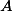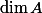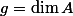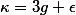# Martin Orr's Blog

## The Masser-Wüstholz period theorem

Posted by Martin Orr on Friday, 30 March 2012 at 12:20

I wanted to write a post about the Masser-Wüstholz isogeny theorem, which gives a quantitative version of Finiteness Theorem I. But it turned out to be too long so for today I will focus on the main ingredient in the proof of the isogeny theorem: the Masser-Wüstholz period theorem.

The period theorem gives a bound for the degree of the smallest abelian subvariety of a fixed abelian varietyhaving a given period ofin its tangent space. In this post I will explain the statement of the period theorem, in particular defining the degree of a (polarised) abelian variety, and give some properties of the degree which will be used in the proof of the isogeny theorem.

Period Theorem. (Masser, Wüstholz 1993) Letbe an abelian variety defined over a number fieldwith a principal polarisation. For any non-zero periodof, the smallest abelian subvarietyofwhose tangent space containssatisfieswhereandare constants depending only on.

Masser and Wüstholz gave a value forofwhere. For myself, I am only interested in the existence of such a bound, but work has been done on improving it. If I correctly understand a recent preprint of Gaudron and Rémond, they show thatsuffices.

## Matlab rant: Elementwise operations on arrays

Posted by Martin Orr on Saturday, 17 March 2012 at 17:27

I have to teach some simple use of Matlab to my engineering students. I don't much like the Matlab language, but then I am unhappy with pretty much every programming language I have ever used except C (which is great for some purposes but they do not include numerical work). Today I am going to rant about the first bit of code in our Matlab course (and probably the first non-trivial bit of code in many Matlab courses and tutorials).

My complaint concerns elementwise operations on arrays (i.e. applying the same operation to every element of an array). These are very important in Matlab, and sometimes you can write code that looks like it is acting on scalars and it just works elementwise on arrays. If scalar code would always just work elementwise on arrays then I might be happy with this (but I worry that if you had such a language, how would you distinguish operations that act on an array as a unit, not just elementwise?).

But sometimes you need to alter things slightly to work elementwise on arrays. I worry that for beginning programmers, the fact that elementwise array operations look so similar to scalar operations makes it difficult to understand when these alterations are required - it would be better to consistently require a syntax meaning "perform this operation elementwise."